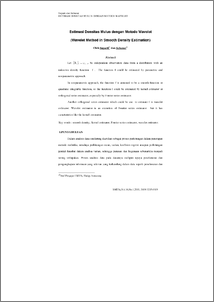# Estimasi Densitas Mulus dengan Metode Wavelet (Wavelet Method in Smooth Density Estimation)

Suparti, Suparti and Subanar, Subanar (2000) Estimasi Densitas Mulus dengan Metode Wavelet (Wavelet Method in Smooth Density Estimation). Jurnal Berkala Ilmiah FMIPA, 10 (1). pp. 91-110. ISSN 0215-9309Preview
PDF
39Kb

Official URL: http://fmipa.ugm.ac.id

## Abstract

Let i = 1,2,…,n be independent observation data from a distribution with an unknown density function f . The function f could be estimated by parametric and nonparametric approach. In nonparametric approach, the function f is assumed to be a smooth function or quadratic integrable function, so the function f could be estimated by kernel estimator or orthogonal series estimator, especially by Fourier series estimator. Another orthogonal series estimator which could be use to estimate f is wavelet estimator. Wavelet estimator is an extention of Fourier series estimator but it has caracteristics like the kernel estimator. Key words : smooth density, kernel estimator, Fourier series estimator, wavelet estimator.

Item Type: Article Q Science > Q Science (General) Faculty of Science and Mathematics > Department of Statistics 3461 INVALID USER 13 Jan 2010 12:48 15 Jan 2010 08:44

Repository Staff Only: item control page